# GeoPandas - PySal, OSM data IO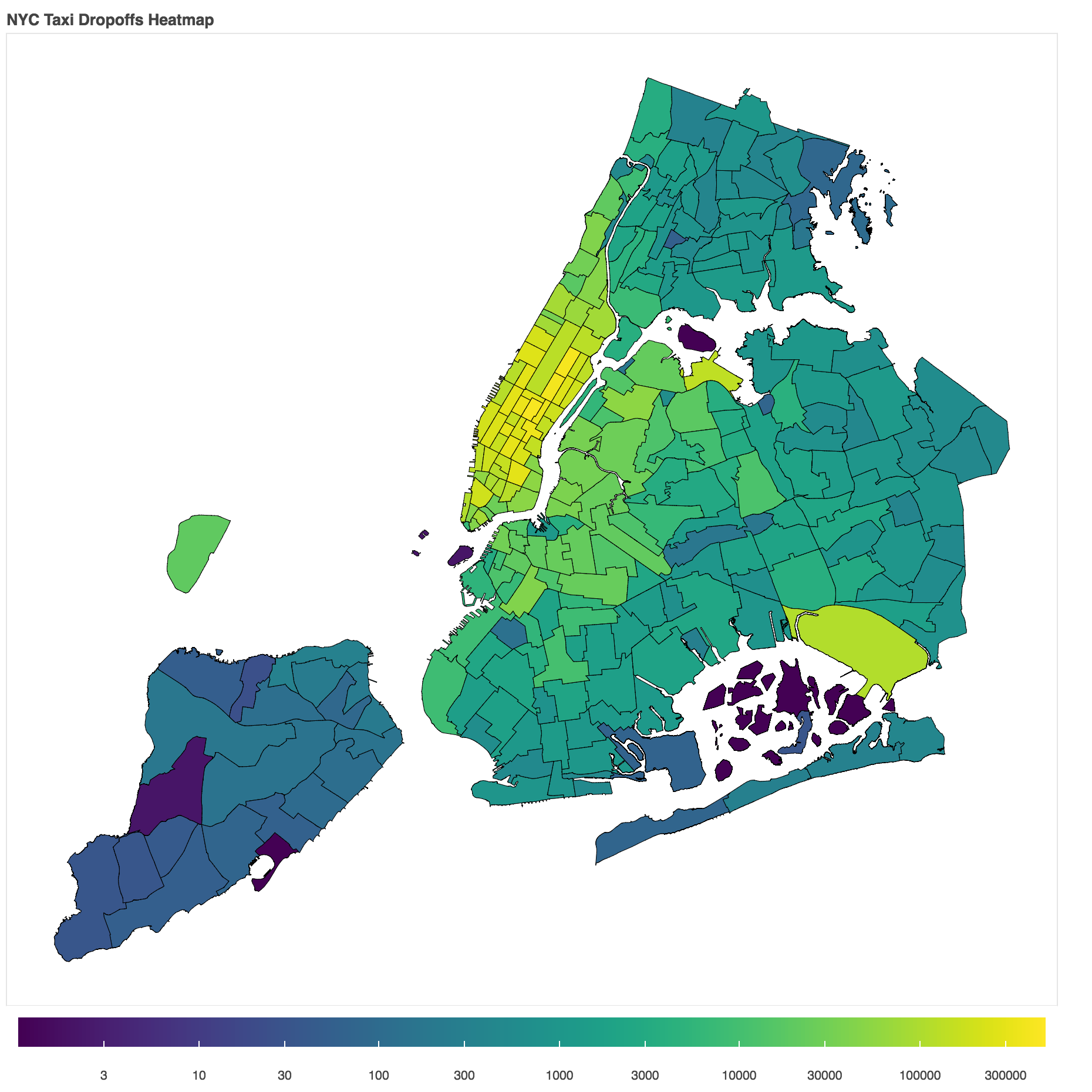Use Pysal to classify data, download vector data from Open Street Maps.

In :
```import geopandas as gpd
import matplotlib.pyplot as plt
%matplotlib inline
```

### Data reclassification¶¶

This is the process of grouping data into set intervals or classes. The class intervals can be manually set or a classification scheme (such as Natural Breaks (Jenks), Quantiles, equal interval..) can be used.

In :
```helsinki_traveltimes = gpd.read_file('./data/travel_times/travel_times_overlay_helsinki.geojson')
```
Out:
car_m_d car_m_t car_r_d car_r_t from_id pt_m_d pt_m_t pt_m_tt pt_r_d pt_r_t pt_r_tt to_id walk_d walk_t GML_ID NAMEFIN NAMESWE NATCODE geometry
0 15981 36 15988 41 6002702 14698 65 73 14698 61 72 5975375 14456 207 27517366 Helsinki Helsingfors 091 POLYGON ((391000.0001349226 6667750.00004299, …
1 16190 34 16197 39 6002701 14661 64 73 14661 60 72 5975375 14419 206 27517366 Helsinki Helsingfors 091 POLYGON ((390750.0001349644 6668000.000042951,…
2 15727 33 15733 37 6001132 14256 59 69 14256 55 62 5975375 14014 200 27517366 Helsinki Helsingfors 091 POLYGON ((391000.0001349143 6668000.000042943,…

The `pt_r_tt` column contains time taken to reach city center. The `walk_d` column is the network distance to city center.

In :
```# remove bad values, no data values
helsinki_traveltimes = helsinki_traveltimes[helsinki_traveltimes['pt_r_tt'] > 0]
```

#### Visualize travel times on map¶¶

In :
```helsinki_traveltimes.plot(column='pt_r_tt', scheme='Fisher_Jenks',
k=9, cmap='RdYlBu', linewidth=0)
# scheme is the classification scheme
# k=9 represents 9 classes in the scheme
# cmap is Red, Yellow, Blue transitions

plt.title('Travel times to city center')
```
Out:
`Text(0.5,1,'Travel times to city center')`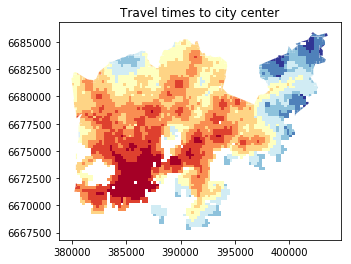In :
```helsinki_traveltimes.plot(column='walk_d', scheme='Fisher_Jenks',
k=9, cmap='RdYlBu', linewidth=0)
# scheme is the classification scheme
# k=9 represents 9 classes in the scheme
# cmap is Red, Yellow, Blue transitions

plt.title('Network distance to city center')
plt.tight_layout()
```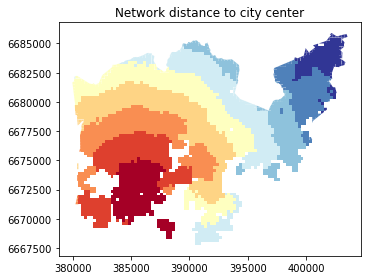### Classify with Pysal¶¶

In :
```import pysal as ps
n_classes = 9
```

Create a classifier object that can be used with `apply()` method of the `DataFrame` object. There are two ways to classify this. One is to send data to Pysal and classify, the other is to get a classifier function and use that to iterate over the rows of the data frame. As you expect, we will do the latter.

The `make()` method of the `Natural_Breaks` class is like a partial constructor. It accepts all parameters of the constructor other than the full data

In :
```nb_classifier = ps.esda.mapclassify.Natural_Breaks.make(k=n_classes)
type(nb_classifier)
```
Out:
`function`
In :
```classification = helsinki_traveltimes[['pt_r_tt']].apply(nb_classifier)
```
In :
```classification.head()
```
Out:
pt_r_tt
0 7
1 7
2 6
3 7
4 7

Thus we have classified the time to city center into `9` classes. The `Natural Breaks` classifier iterates over the whole data making `n_classes` groups such that the variability within groups is minimum and variability between groups is higher.

In :
```# rename the column
classification.rename(columns={'pt_r_tt':'nb_pt_r_tt'}, inplace=True)
```
In :
```classification.head()
```
Out:
nb_pt_r_tt
0 7
1 7
2 6
3 7
4 7

Join with original geodataframe

In :
```helsinki_traveltimes.join(classification, how='outer')
```
Out:
car_m_d car_m_t car_r_d car_r_t from_id pt_m_d pt_m_t pt_m_tt pt_r_d pt_r_t pt_r_tt to_id walk_d walk_t GML_ID NAMEFIN NAMESWE NATCODE geometry
0 15981 36 15988 41 6002702 14698 65 73 14698 61 72 5975375 14456 207 27517366 Helsinki Helsingfors 091 POLYGON ((391000.0001349226 6667750.00004299, …
1 16190 34 16197 39 6002701 14661 64 73 14661 60 72 5975375 14419 206 27517366 Helsinki Helsingfors 091 POLYGON ((390750.0001349644 6668000.000042951,…
2 15727 33 15733 37 6001132 14256 59 69 14256 55 62 5975375 14014 200 27517366 Helsinki Helsingfors 091 POLYGON ((391000.0001349143 6668000.000042943,…
3 15975 33 15982 37 6001131 14512 62 73 14512 58 70 5975375 14270 204 27517366 Helsinki Helsingfors 091 POLYGON ((390750.0001349644 6668000.000042951,…
4 16136 35 16143 40 6001138 14730 65 73 14730 61 72 5975375 14212 203 27517366 Helsinki Helsingfors 091 POLYGON ((392500.0001346234 6668000.000042901,…
In :
```helsinki_traveltimes.columns
```
Out:
```Index(['car_m_d', 'car_m_t', 'car_r_d', 'car_r_t', 'from_id', 'pt_m_d',
'pt_m_t', 'pt_m_tt', 'pt_r_d', 'pt_r_t', 'pt_r_tt', 'to_id', 'walk_d',
'walk_t', 'GML_ID', 'NAMEFIN', 'NAMESWE', 'NATCODE', 'geometry'],
dtype='object')```

#### Custom classifier¶¶

We can define our own classifier as a function and do the rest as in regular pandas

In :
```# find places that are within 20 mins of drive time, but at least 5 KM away from city
# center
def binary_classifier(row):
if row['pt_r_tt'] <= 20 and row['walk_d'] >= 4000:
return 1
else:
return 0
```
In :
```# time this classification
%time

# apply the classifier
helsinki_traveltimes['suitable_grid'] = helsinki_traveltimes.apply(binary_classifier, axis=1)

# see output
```
```CPU times: user 4 µs, sys: 1e+03 ns, total: 5 µs
Wall time: 8.82 µs
```
Out:
car_m_d car_m_t car_r_d car_r_t from_id pt_m_d pt_m_t pt_m_tt pt_r_d pt_r_t pt_r_tt to_id walk_d walk_t GML_ID NAMEFIN NAMESWE NATCODE geometry suitable_grid
0 15981 36 15988 41 6002702 14698 65 73 14698 61 72 5975375 14456 207 27517366 Helsinki Helsingfors 091 POLYGON ((391000.0001349226 6667750.00004299, … 0
1 16190 34 16197 39 6002701 14661 64 73 14661 60 72 5975375 14419 206 27517366 Helsinki Helsingfors 091 POLYGON ((390750.0001349644 6668000.000042951,… 0
2 15727 33 15733 37 6001132 14256 59 69 14256 55 62 5975375 14014 200 27517366 Helsinki Helsingfors 091 POLYGON ((391000.0001349143 6668000.000042943,… 0
3 15975 33 15982 37 6001131 14512 62 73 14512 58 70 5975375 14270 204 27517366 Helsinki Helsingfors 091 POLYGON ((390750.0001349644 6668000.000042951,… 0
4 16136 35 16143 40 6001138 14730 65 73 14730 61 72 5975375 14212 203 27517366 Helsinki Helsingfors 091 POLYGON ((392500.0001346234 6668000.000042901,… 0
In :
```helsinki_traveltimes.shape
```
Out:
`(3816, 20)`
In :
```helsinki_traveltimes['suitable_grid'].value_counts()
```
Out:
```0    3794
1      22
Name: suitable_grid, dtype: int64```
In :
```helsinki_traveltimes.plot(column='suitable_grid')
```
Out:
`<matplotlib.axes._subplots.AxesSubplot at 0x10e1cfe80>`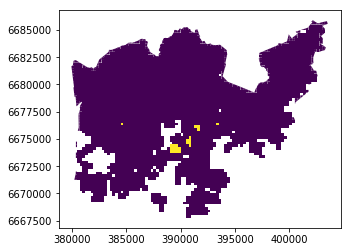Use OSMnx library to download vector, network data from open streets maps server.

In :
```import osmnx as ox
chennai_graph = ox.graph_from_place('Chennai, Tamil Nadu, India')
type(chennai_graph)
```
Out:
`networkx.classes.multidigraph.MultiDiGraph`
In :
```ox.plot_graph(chennai_graph)
```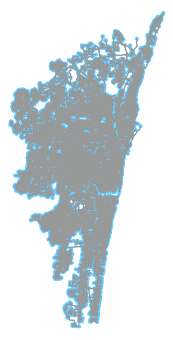Out:
```(<Figure size 216.459x432 with 1 Axes>,
<matplotlib.axes._subplots.AxesSubplot at 0x1542a5ecf8>)```

Chennai is quite large and it takes a long time. Let us try an address. OSMnx will apply a bound box around it and get the network around it.

In :
```redlands_graph = ox.graph_from_address('380 New York St, Redlands, CA, USA')
```
In :
```ox.plot_graph_folium(redlands_graph)
```
Out: